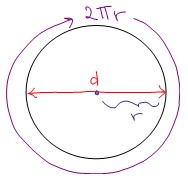Ontario Tech acknowledges the lands and people of the Mississaugas of Scugog Island First Nation.

We are thankful to be welcome on these lands in friendship. The lands we are situated on are covered by the Williams Treaties and are the traditional territory of the Mississaugas, a branch of the greater Anishinaabeg Nation, including Algonquin, Ojibway, Odawa and Pottawatomi. These lands remain home to many Indigenous nations and peoples.

We acknowledge this land out of respect for the Indigenous nations who have cared for Turtle Island, also called North America, from before the arrival of settler peoples until this day. Most importantly, we acknowledge that the history of these lands has been tainted by poor treatment and a lack of friendship with the First Nations who call them home.

This history is something we are all affected by because we are all treaty people in Canada. We all have a shared history to reflect on, and each of us is affected by this history in different ways. Our past defines our present, but if we move forward as friends and allies, then it does not have to define our future.

# Circles

## INTRODUCTION

Here are a few quick facts that are useful to know about a circle that has radius r:Diameter = 2r
Circumference = 2π r
Area = πr2

Example:
If a circle has an area of 16π, what is its radius?

Solution:

$$\displaylines{A = \pi r^2~ \text{but we have} ~A=16\pi ~\text{so} ~\pi r^2 = 16\pi \\ r^2 = 16 \\ r = 4}$$

## THE EQUATION OF A CIRCLE

A circle with radius r centred at the origin has the equation:

$$x^2+y^2=r^2$$

A circle whose centre is at the point (a,b) has the equation:

$$(x-a)^2+(y-b)^2=r^2$$

Example: Consider the circle given by the equation  $$(x - 3)^2 + (y + 2)^2 = 49$$

What is the position of it's centre? What is the radius?

Solution:

The centre is at $$(3, -2)$$.